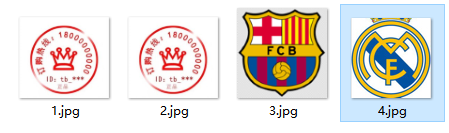# 【python 图片搜索】python 快速计算两个图片的相似度

• 相似图片搜索
• 图片相似度比较
• 百度识图
• 图片比较

Step1.缩小尺寸

Step2.灰度处理

Step3.计算平均值

Step4.计算哈希

Step5.对比哈希


# -*- coding:utf-8 -*-

from functools import reduce
from PIL import Image

# 计算图片的局部哈希值--pHash
def phash(img):
"""
:param img: 图片
:return: 返回图片的局部hash值
"""
img = img.resize((8, 8), Image.ANTIALIAS).convert('L')
avg = reduce(lambda x, y: x + y, img.getdata()) / 64.
hash_value=reduce(lambda x, y: x | (y << y), enumerate(map(lambda i: 0 if i < avg else 1, img.getdata())), 0)
print(hash_value)
return hash_value

# 计算汉明距离:
def hamming_distance(a, b):
"""
:param a: 图片1的hash值
:param b: 图片2的hash值
:return: 返回两个图片hash值的汉明距离
"""
hm_distance=bin(a ^ b).count('1')
print(hm_distance)
return hm_distance

# 计算两个图片是否相似:
def is_imgs_similar(img1,img2):
"""
:param img1: 图片1
:param img2: 图片2
:return:  True 图片相似  False 图片不相似
"""
return True if hamming_distance(phash(img1),phash(img2)) <= 5 else False

if __name__ == '__main__':

# 读取图片
sensitive_pic = Image.open("3.jpg")
target_pic = Image.open("4.jpg")

# 比较图片相似度
result=is_imgs_similar(target_pic, sensitive_pic)

print(result)15824809348783249859
18411139146200482779
24
False

Process finished with exit code 0


14384397865107178495
14384397865107178495
0
True

Process finished with exit code 0


# -*- encoding=utf-8 -*-

from functools import reduce
from PIL import Image

# 这种算法的优点是简单快速，不受图片大小缩放的影响，
# 缺点是图片的内容不能变更。如果在图片上加几个文字，它就认不出来了。
# 所以，它的最佳用途是根据缩略图，找出原图。

# 计算图片的局部哈希值--pHash
def phash(img):
"""
:param img: 图片
:return: 返回图片的局部hash值
"""
img = img.resize((8, 8), Image.ANTIALIAS).convert('L')
avg = reduce(lambda x, y: x + y, img.getdata()) / 64.
hash_value=reduce(lambda x, y: x | (y << y), enumerate(map(lambda i: 0 if i < avg else 1, img.getdata())), 0)

return hash_value

# 自定义计算两个图片相似度函数局部敏感哈希算法
def phash_img_similarity(img1_path,img2_path):
"""
:param img1_path: 图片1路径
:param img2_path: 图片2路径
:return: 图片相似度
"""

# 读取图片
img1 = Image.open(img1_path)
img2 = Image.open(img2_path)

# 计算两个图片的局部哈希值

# 计算局部敏感哈希值
img1_phash = str(phash(img1))
img2_phash = str(phash(img2))

# 打印局部敏感哈希值
print(img1_phash)
print(img2_phash)

# 计算汉明距离

distance = bin(phash(img1) ^ phash(img2)).count('1')
print(distance)

print(max(len(bin(phash(img1))), len(bin(phash(img1)))))

similary = 1 - distance / max(len(bin(phash(img1))), len(bin(phash(img1))))
print("两张图片相似度为:%s" % similary)

if __name__ == '__main__':
img1_path = r'F:\img_spam\test\3.png'
img2_path = r'F:\img_spam\test\4.png'
similary = phash_img_similarity(img1_path, img2_path)

E:\laidefa\python.exe F:/文本标签/图片反垃圾/感知哈希算法计算图片相似度.py
18446604991956385279
18446604991956385279
0
66

Process finished with exit code 0


09-2308-295938
04-241万+
12-143万+
07-081万+
02-21826
09-131044
07-121万+
06-076887
12-07909
08-26728
09-22
03-021万+
04-094988
02-161959
03-24430
02-156713
04-247万+
09-176万+